# Image Approximation with Fourier and Wavelets¶

Important: Please read the installation page for details about how to install the toolboxes. $\newcommand{\dotp}{\langle #1, #2 \rangle}$ $\newcommand{\enscond}{\lbrace #1, #2 \rbrace}$ $\newcommand{\pd}{ \frac{ \partial #1}{\partial #2} }$ $\newcommand{\umin}{\underset{#1}{\min}\;}$ $\newcommand{\umax}{\underset{#1}{\max}\;}$ $\newcommand{\umin}{\underset{#1}{\min}\;}$ $\newcommand{\uargmin}{\underset{#1}{argmin}\;}$ $\newcommand{\norm}{\|#1\|}$ $\newcommand{\abs}{\left|#1\right|}$ $\newcommand{\choice}{ \left\{ \begin{array}{l} #1 \end{array} \right. }$ $\newcommand{\pa}{\left(#1\right)}$ $\newcommand{\diag}{{diag}\left( #1 \right)}$ $\newcommand{\qandq}{\quad\text{and}\quad}$ $\newcommand{\qwhereq}{\quad\text{where}\quad}$ $\newcommand{\qifq}{ \quad \text{if} \quad }$ $\newcommand{\qarrq}{ \quad \Longrightarrow \quad }$ $\newcommand{\ZZ}{\mathbb{Z}}$ $\newcommand{\CC}{\mathbb{C}}$ $\newcommand{\RR}{\mathbb{R}}$ $\newcommand{\EE}{\mathbb{E}}$ $\newcommand{\Zz}{\mathcal{Z}}$ $\newcommand{\Ww}{\mathcal{W}}$ $\newcommand{\Vv}{\mathcal{V}}$ $\newcommand{\Nn}{\mathcal{N}}$ $\newcommand{\NN}{\mathcal{N}}$ $\newcommand{\Hh}{\mathcal{H}}$ $\newcommand{\Bb}{\mathcal{B}}$ $\newcommand{\Ee}{\mathcal{E}}$ $\newcommand{\Cc}{\mathcal{C}}$ $\newcommand{\Gg}{\mathcal{G}}$ $\newcommand{\Ss}{\mathcal{S}}$ $\newcommand{\Pp}{\mathcal{P}}$ $\newcommand{\Ff}{\mathcal{F}}$ $\newcommand{\Xx}{\mathcal{X}}$ $\newcommand{\Mm}{\mathcal{M}}$ $\newcommand{\Ii}{\mathcal{I}}$ $\newcommand{\Dd}{\mathcal{D}}$ $\newcommand{\Ll}{\mathcal{L}}$ $\newcommand{\Tt}{\mathcal{T}}$ $\newcommand{\si}{\sigma}$ $\newcommand{\al}{\alpha}$ $\newcommand{\la}{\lambda}$ $\newcommand{\ga}{\gamma}$ $\newcommand{\Ga}{\Gamma}$ $\newcommand{\La}{\Lambda}$ $\newcommand{\si}{\sigma}$ $\newcommand{\Si}{\Sigma}$ $\newcommand{\be}{\beta}$ $\newcommand{\de}{\delta}$ $\newcommand{\De}{\Delta}$ $\newcommand{\phi}{\varphi}$ $\newcommand{\th}{\theta}$ $\newcommand{\om}{\omega}$ $\newcommand{\Om}{\Omega}$

This numerical tour overviews the use of Fourier and wavelets for image approximation.

In :
addpath('toolbox_signal')


Note: to measure the error of an image $f$ with its approximation $f_M$, we use the SNR measure, defined as $$\text{SNR}(f,f_M) = -20\log_{10} \pa{ \frac{ \norm{f-f_M} }{ \norm{f} } },$$ which is a quantity expressed in decibels (dB). The higer the SNR, the better the quality.

First we load an image $f \in \RR^N$ of $N = N_0 \times N_0$ pixels.

In :
name = 'lena';
n0 = 512;


Display the original image.

In :
clf;
imageplot( f, 'Image f');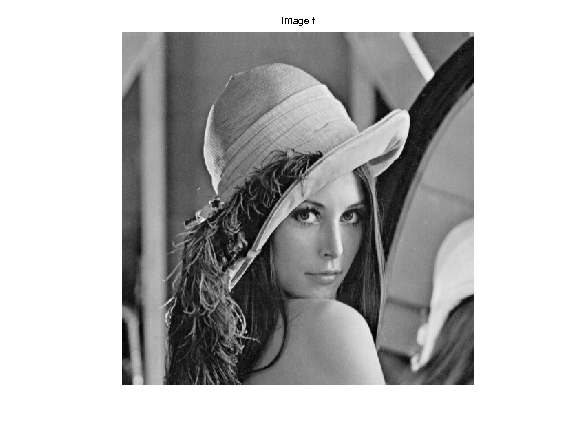Display a zoom in the middle.

In :
clf;
imageplot( crop(f,64), 'Zoom' );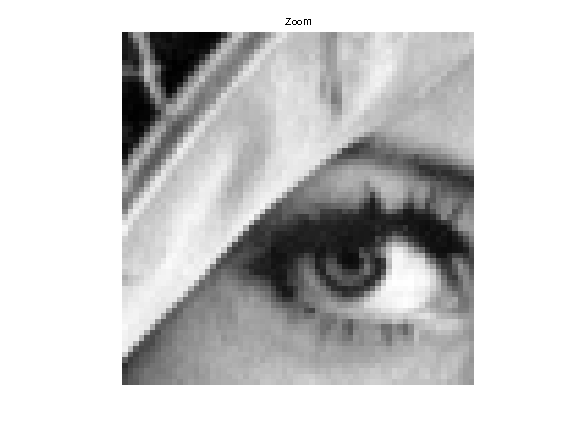An image is a 2D array, that can be modified as a matrix.

In :
clf;
imageplot(-f, '-f', 1,2,1);
imageplot(f(n0:-1:1,:), 'Flipped', 1,2,2);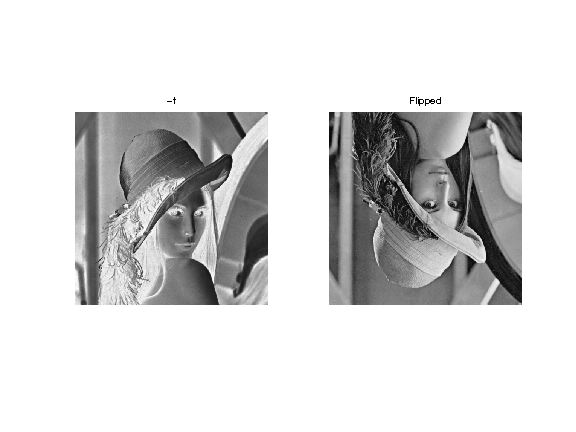Blurring is achieved by computing a convolution $f \star h$ with a kernel $h$.

Compute the low pass kernel.

In :
k = 9; % size of the kernel
h = ones(k,k);
h = h/sum(h(:)); % normalize


Compute the convolution $f \star h$.

In :
fh = perform_convolution(f,h);


Display.

In :
clf;
imageplot(fh, 'Blurred image');## Fourier Transform¶

The Fourier orthonormal basis is defined as $$\psi_m(k) = \frac{1}{\sqrt{N}}e^{\frac{2i\pi}{N_0} \dotp{m}{k} }$$ where $0 \leq k_1,k_2 < N_0$ are position indexes, and $0 \leq m_1,m_2 < N_0$ are frequency indexes.

The Fourier transform $\hat f$ is the projection of the image on this Fourier basis $$\hat f(m) = \dotp{f}{\psi_m}.$$

The Fourier transform is computed in $O(N \log(N))$ operation using the FFT algorithm (Fast Fourier Transform). Note the normalization by $\sqrt{N}=N_0$ to make the transform orthonormal.

In :
F = fft2(f) / n0;


We check this conservation of the energy.

In :
disp(strcat(['Energy of Image:   ' num2str(norm(f(:)))]));
disp(strcat(['Energy of Fourier: ' num2str(norm(F(:)))]));

Energy of Image:   255.9831
Energy of Fourier: 255.9831


Compute the logarithm of the Fourier magnitude $\log(\abs{\hat f(m)} + \epsilon)$, for some small $\epsilon$.

In :
L = fftshift(log( abs(F)+1e-1 ));


Display. Note that we use the function |fftshift| is useful to put the 0 low frequency in the middle.

In :
clf;
imageplot(L, 'Log(Fourier transform)');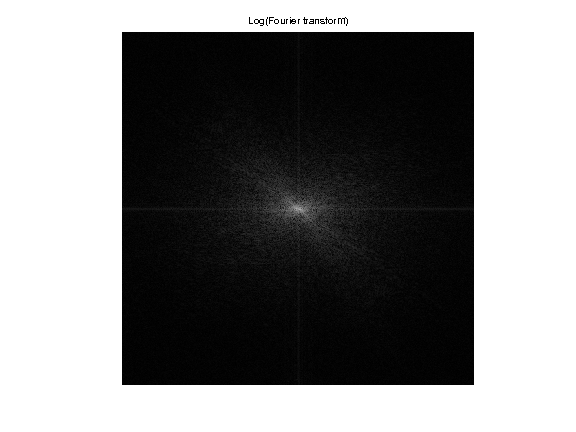## Linear Fourier Approximation¶

An approximation is obtained by retaining a certain set of index $I_M$ $$f_M = \sum_{ m \in I_M } \dotp{f}{\psi_m} \psi_m.$$

Linear approximation is obtained by retaining a fixed set $I_M$ of $M = \abs{I_M}$ coefficients. The important point is that $I_M$ does not depend on the image $f$ to be approximated.

For the Fourier transform, a low pass linear approximation is obtained by keeping only the frequencies within a square. $$I_M = \enscond{m=(m_1,m_2)}{ -q/2 \leq m_1,m_2 < q/2 }$$ where $q = \sqrt{M}$.

This can be achieved by computing the Fourier transform, setting to zero the $N-M$ coefficients outside the square $I_M$ and then inverting the Fourier transform.

Number $M$ of kept coefficients.

In :
M = n0^2/64;


Exercise 1

Perform the linear Fourier approximation with $M$ coefficients. Store the result in the variable |fM|. isplay

In :
exo1()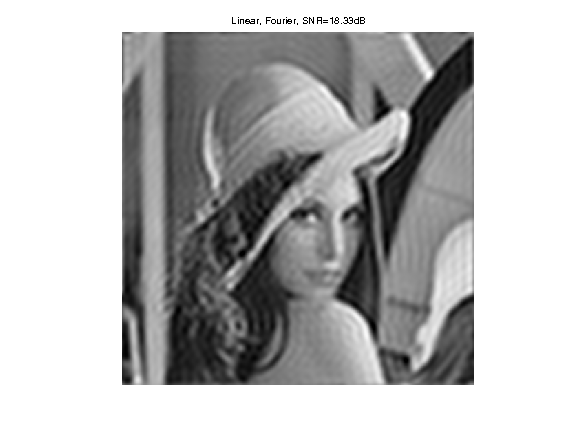In :
%% Insert your code here.


Compare two 1D profile (lines of the image). This shows the strong ringing artifact of the linea approximation.

In :
clf;
subplot(2,1,1);
plot(f(:,n0/2));
axis('tight'); title('f');
subplot(2,1,2);
plot(fM(:,n0/2));
axis('tight'); title('f_M');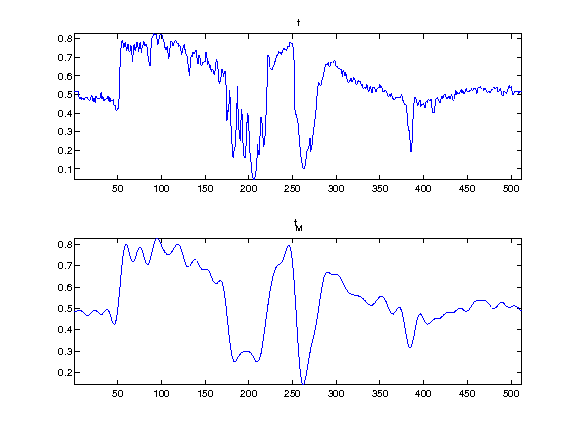## Non-linear Fourier Approximation¶

Non-linear approximation is obtained by keeping the $M$ largest coefficients. This is equivalently computed using a thresholding of the coefficients $$I_M = \enscond{m}{ \abs{\dotp{f}{\psi_m}}>T }.$$

Set a threshold $T>0$.

In :
T = .2;


Compute the Fourier transform.

In :
F = fft2(f) / n0;


Do the hard thresholding.

In :
FT = F .* (abs(F)>T);


Display. Note that we use the function |fftshift| is useful to put the 0 low frequency in the middle.

In :
clf;
L = fftshift(log( abs(FT)+1e-1 ));
imageplot(L, 'thresholded Log(Fourier transform)');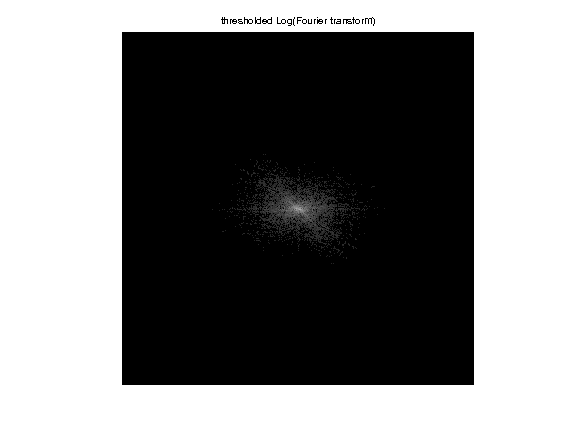Inverse Fourier transform to obtained $f_M$

In :
fM = real( ifft2(FT)*n0 );


Display.

In :
clf;
imageplot(clamp(fM), ['Non-linear, Fourier, SNR=' num2str(snr(f,fM), 4) 'dB']);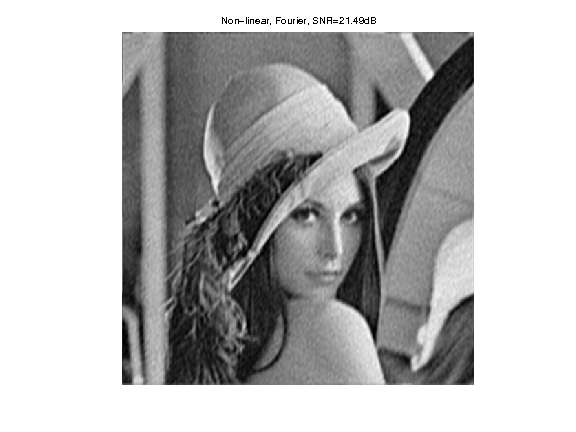Given a $T$, the number of coefficients is obtained by counting the non thresholded coefficients $\abs{I_M}$.

In :
m = sum(FT(:)~=0);
disp(['M/N = 1/'  num2str(round(n0^2/m)) '.']);

M/N = 1/32.


Exercise 2

Compute the value of the threshold $T$ so that the number of coefficients is $M$. Display the corresponding approximation $f_M$. isplay

In :
exo2()